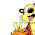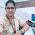## Monday, May 9, 2011

### Multiple Y-axis in a R plot

I often have to to plot multiple time-series with different scale of values for comparative purposes, and although placing them in different rows are useful, placing on a same graph is still useful sometimes...
I searched a bit about this, and found some nice suggestions for 2 Y-axis, but haven't found anything for more 2+. So here's my solution:

#Create Dataset

time<-seq(7000,3400,-200)
pop<-c(200,400,450,500,300,100,400,700,830,1200,400,350,200,700,370,800,200,100,120)
grp<-c(2,5,8,3,2,2,4,7,9,4,4,2,2,7,5,12,5,4,4)
med<-c(1.2,1.3,1.2,0.9,2.1,1.4,2.9,3.4,2.1,1.1,1.2,1.5,1.2,0.9,0.5,3.3,2.2,1.1,1.2)

#Define Margins. The trick is to use give as much space possible on the left margin (second value)
par(mar=c(5, 12, 4, 4) + 0.1)

#Plot the first time series. Notice that you don't have to draw the axis nor the labels

plot(time, pop, axes=F, ylim=c(0,max(pop)), xlab="", ylab="",type="l",col="black", main="",xlim=c(7000,3400))
points(time,pop,pch=20,col="black")
axis(2, ylim=c(0,max(pop)),col="black",lwd=2)
mtext(2,text="Population",line=2)

#Plot the second time series. The command par(new=T) is handy here. If you just need to plot two timeseries, you could also use the right vertical axis as well. In that case you have to substitute "2" with "4" in the functions axis() and mtext(). Notice that in both functions lines is increased so that the new axis and its label is placed to the left of the first one. You don't need to increase the value if you use the right vertical axis.

par(new=T)
plot(time, med, axes=F, ylim=c(0,max(med)), xlab="", ylab="",
type="l",lty=2, main="",xlim=c(7000,3400),lwd=2)
axis(2, ylim=c(0,max(med)),lwd=2,line=3.5)
points(time, med,pch=20)
mtext(2,text="Median Group Size",line=5.5)

#Plot the third time series. Again the line parameter are both further increased.

par(new=T)
plot(time, grp, axes=F, ylim=c(0,max(grp)), xlab="", ylab="",
type="l",lty=3, main="",xlim=c(7000,3400),lwd=2)
axis(2, ylim=c(0,max(grp)),lwd=2,line=7)

points(time, grp,pch=20)
mtext(2,text="Number of Groups",line=9)

#We can now draw the X-axis, which is of course shared by all the three time-series.

axis(1,pretty(range(time),10))
mtext("cal BP",side=1,col="black",line=2)

#And then plot the legend.

legend(x=7000,y=12,legend=c("Population","Median Group Size","Number of Groups"),lty=c(1,2,3))

1.Great post, I liked the creative use of Par(New=T) combined with left margin space to depict scales of different series.
Is it possible to add a background grey grid to this plot, as I would like to use this framework for some of my plots. thnks and rgds, snvk

2.Thanks! You can change the background color using par(bg="lightgrey"), and possible add a grid using the function...well..grid(), which allows you to define the number of rows and columns. A longer but perhaps more elegant option is to use abline().
E.

3.Hi! Thanks for writing this post. But when I try the commands, I am getting the different axes but the graph is not plotted. Can you tell me what I am missing?

4.Hi! thanks for the post, it has been very usefull. I only have one more question. As you can see your 2nd Y-axis (Median Groupe Size) is a little longer than the other two. How coul I fix it? I've tried it modifying ylim but I'm not able to get the result that I'm looking for.

Nerea

5.@ Aparna, that's odd....does it give you an error message?

6.@Nerea, I think you could use the parameter "yaxp" inside the function axis(). For example if you type:

plot(time, med, axes=F, ylim=c(0,3.5), xlab="", ylab="",
type="l",lty=2, main="",xlim=c(7000,3400),lwd=2)
axis(2, yaxp=c(0,3.5,7),lwd=2,line=3.5)

it should work...

1.It works!

Thanks again!

7.Hey, Thank you so much! Your example has been the most helpful for me trying to figure out how to put multiple Y axes over the same X axis. Much appreciated.

8.Thanks for this post, it is very helpful. Is it possible to do this but with different x values as well? I have two sets of timeseries data, so I still only want one x-axis (time), but the 2 datasets are different lengths. Is there a modification of your method that would allow this? Many thanks.

9.Thanks very much for the useful post. I am trying to achieve exactly the same result, only this time with "population" mapped to a bar chart with bar plot(), seems impossible to align the points along the line plots (e.g. from Number of groups in this example) to the midpoints of the bars from the population bar plot. How can I do this?

10.Hi thanks for this little manual, one problem I´m facing right now is that the second y-axis is paionted outside of the plotting area..is there a way to automatically adjust the scale?

11.i am unable to run the script on my data.. anyone please help.

DO (mg/l) EC (mmho/cm) TC (MPN/100 ml)
7.7 312.0 8740
6.9 324.5 12700
7.1 282.5 16200
7.7 271.5 14700
7.3 268.5 29700
7.2 311.5 37500
9.3 397.5 17500
8.8 348.6 11700
6.4 311.1 12700
6.8 349.1 13500
6.6 171.0 5500
6.7 260.0 9500
7.7 275.0 1480
7.6 349.0 17000
6.2 300.0 8400
8.0 265.0 24000
7.3 278.0 5400
7.3 259.0 54000
7.1 364.0 21000
11.5 431.0 14000
6.1 266.1 9400
6.7 356.1 16000
6.8 342.0 11000
6.2 325.3 8477
6.2 321.2 8372
6.4 333.9 8702
6.5 337.5 8795
6.4 336.0 8756
6.6 342.0 8912
6.6 343.3 8947
6.6 344.2 8970
6.6 346.1 9021
6.2 323.5 8430
6.5 338.8 8830
6.3 326.5 8508
6.3 327.1 8523
6.2 323.5 8430
6.1 319.0 8313
6.7 348.8 9090
9.5 182.0 2100

12.Many Thanks for your wonderful post. I can plot 3 Y axis like you. When I plot 4th, it is redrawn on same 3rd Y axis. I had modified par giving more space for left margin. However still 4th Y axis redraws on 3rd. Would like to know the solution.